# getspanel: Getting Started

The `getspanel` package can be downloaded and installed from CRAN here by simply using:

``install.packages("getspanel")``

The source code of the package is on GitHub and the development version can be installed using:

``````# install.packages("devtools")
devtools::install_github("moritzpschwarz/getspanel", ref = "devel")``````

Once installed we need to load the library:

``library(getspanel)``

Currently the package is called getspanel to align with the gets package, but it’s main function of course remains the isatpanel function.

The isatpanel function implements the empirical break detection algorithm that is described in a paper by Felix Pretis and Moritz Schwarz and was applied to a study by Nico Koch and colleagues on EU Road CO2 emissions, which was published in Nature Energy in 2022.

A quick overview over what has changed:

• We can now use the function approach as well as the traditional gets approach. This means that we can specify a model using `y` and `mxreg` as well as `time` and `id` as vectors, but we can now also simply supply a `data.frame` and a `function` in the form `y ~ x + z + I(x^2)` to e.g. specify polynomials. This means we will then need an `index` argument, which specifies the

• The `ar` argument now works

• We can now use the `fixest` package to speed up model estimation with large `i` (for short panels, the default method is still faster).The package can be activated using the new `engine` argument.

• Using the `fixest` package also allows us to calculate clustered standard errors.

• We can now be certain that unbalanced panels would work as intended, which was not the case before.

• The `mxbreak` and `break.method` arguments have been removed. Instead the function now produces the break matrix itself. This now implements the following saturation methods in a user friendly way:

• iis: Impulse Indicator Saturation

• jsis: Joint Step Indicator Saturation (Common Breaks over time)

• csis: Coefficient Step Indicator Saturation (Common Coefficient Breaks over time)

• fesis: Fixed Effect Step Indicator Saturation (Breaks in the Group Fixed Effect over time)

• cfesis: Coefficient Fixed Effect Step Indicator Saturation (Breaks in the coefficient for each individual)

# The isatpanel function

We first load some data of EU CO2 Emissions in the housing sector.

``````data("EUCO2residential")
# A tibble: 6 × 9
country  year  lgdp  lhdd  lcdd urban av.rate     pop agg.directem
<chr>   <dbl> <dbl> <dbl> <dbl> <dbl>   <dbl>   <dbl>        <dbl>
1 Austria  1969  25.6    NA    NA  65.2      NA      NA           NA
2 Austria  1970  25.7    NA    NA  65.3      NA      NA           NA
3 Austria  1971  25.8    NA    NA  65.3      NA 7500482           NA
4 Austria  1972  25.8    NA    NA  65.3      NA 7544201           NA
5 Austria  1973  25.9    NA    NA  65.3      NA 7586115           NA
6 Austria  1974  25.9    NA    NA  65.3      NA 7599038           NA

# let's subset this a little bit to speed this up
EUCO2residential <- EUCO2residential[EUCO2residential\$year > 2000 &
EUCO2residential\$country %in% c("Germany", "Austria",
"Belgium", "Italy",
"Sweden", "Denmark"),]

# let's create a log emissions per capita variable
EUCO2residential\$lagg.directem_pc <- log(EUCO2residential\$agg.directem/EUCO2residential\$pop)

# and let's also turn off printing the intermediate output from isatpanel
options(print.searchoutput = FALSE)``````

Let’s look at how we input what we want to model. Each `isatpanel` command takes:

## Basics

• A specification of the source data, the group and time variable and the group-time characteristics. This can be entered into the function in two ways:
1. In the gets package style i.e. using vectors and matrices to specify `y`, `mxreg`, `time` and `id`

2. But also in a form that resembles the `lm` and `plm` specification i.e. inputting a `data.frame` (or `matrix` or `tibble`), a `formula` argument as well as character vectors for `index` (in the form `c("group_variable_name", "time_variable_name")`)

• A an argument for the Fixed Effect Specification using `effect`.

This already means that the following two commands will give the same result:

Using the new method

``````is_lm <- isatpanel(data = EUCO2residential,
formula = lagg.directem_pc ~ lgdp + I(lgdp^2) + pop,
index = c("country","year"),

effect = "twoways",

fesis = TRUE)``````

``````is_gets <- isatpanel(y = EUCO2residential\$lagg.directem_pc,
mxreg = EUCO2residential\$lgdp,
time = EUCO2residential\$year,
id = EUCO2residential\$country,

effect = "twoways",

fesis = TRUE)``````

From here onwards, I will use the `lm` notation.

## Plotting

We can plot these simply using the default plotting methods (rely on the ggplot2 package):

``plot(is_lm)``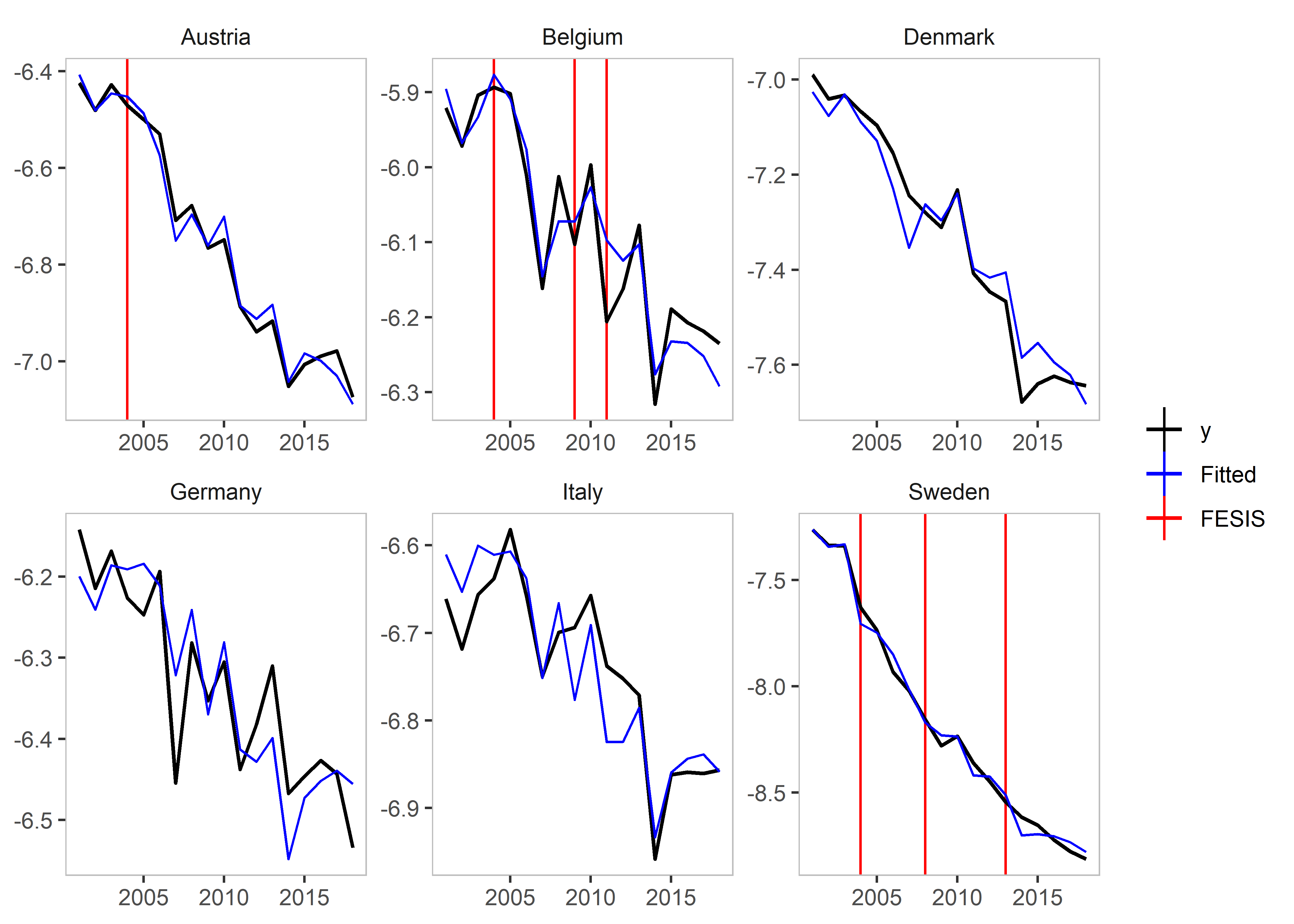``plot_grid(is_lm)``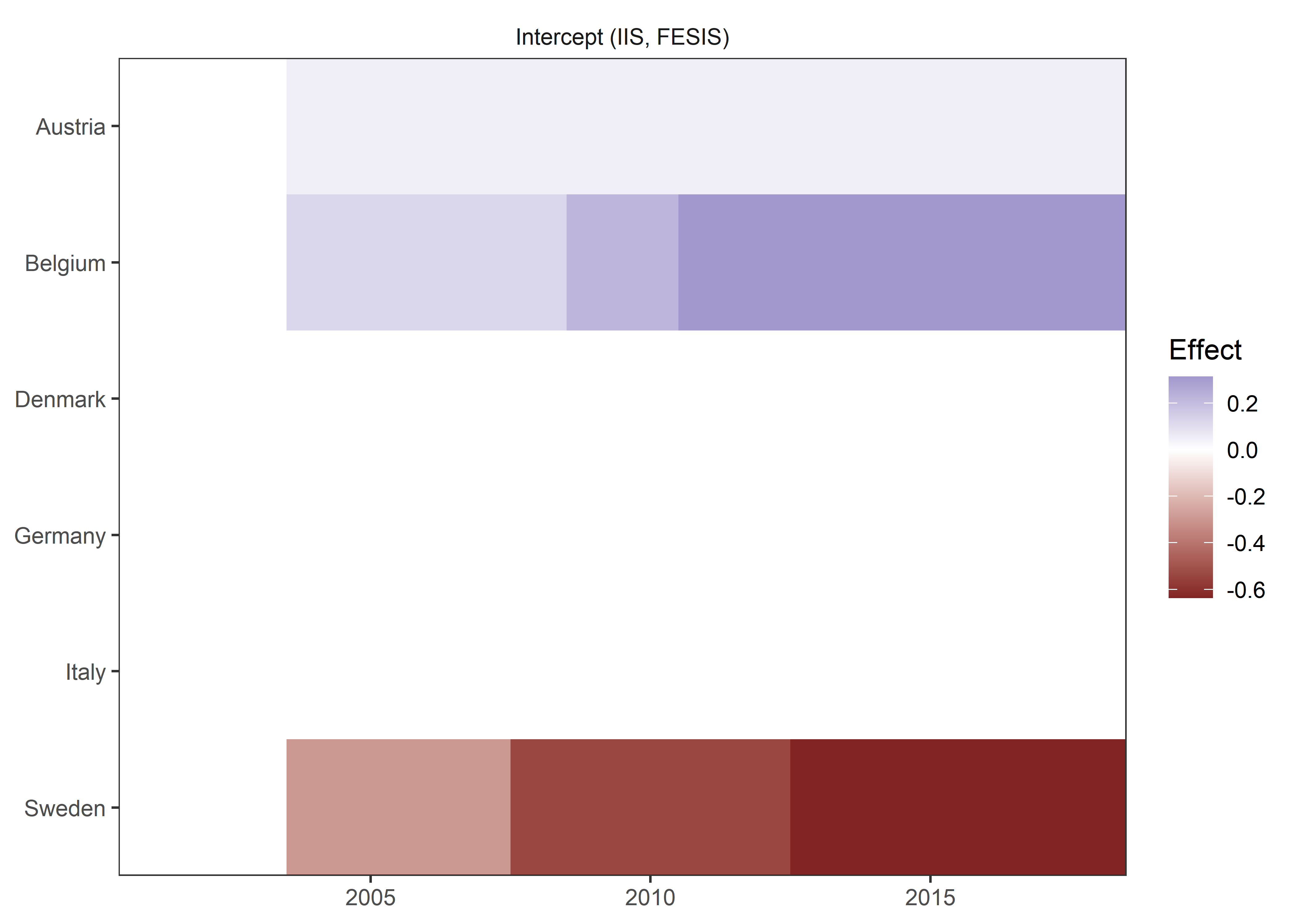``plot_counterfactual(is_lm)``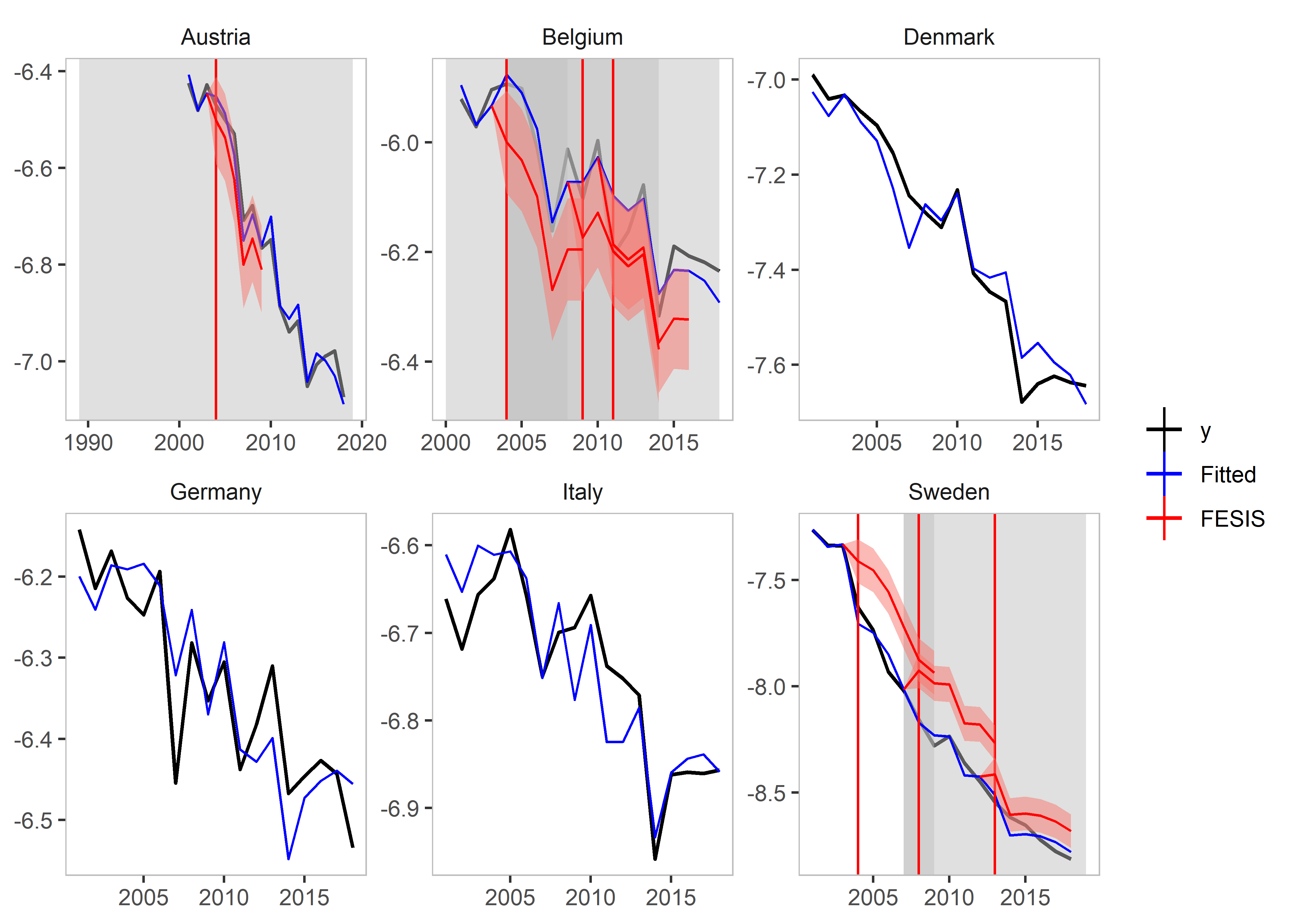## Saturation Methods

### Impulse Indicator Saturation

This argument works just as in the gets package. The method simply adds a `0` and `1` dummy for each observation.

Simply set `iis = TRUE`.

``````iis_example <- isatpanel(data = EUCO2residential,
formula = lagg.directem_pc ~ lgdp + I(lgdp^2) + pop,
index = c("country","year"),

effect = "twoways",

iis = TRUE,
fesis = TRUE)``````
``plot(iis_example)``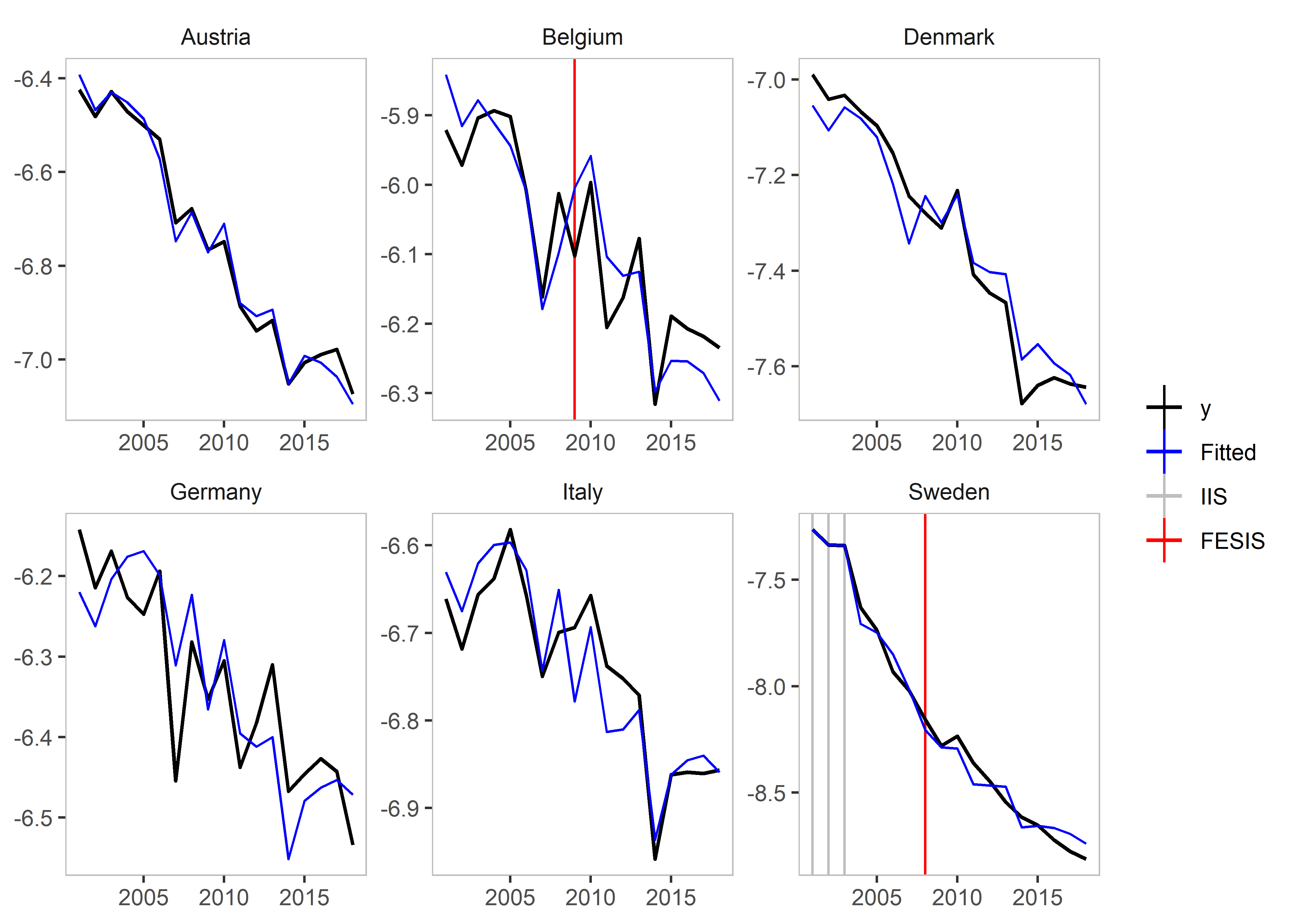### Step Indicator Saturation

Traditional Step Indicator Saturation does not make sense in a panel setting. Therefore, the gets function of `sis` is disabled.

### Joint Step Indicator Saturation

It is possible, however, to consider Step Indicator Saturation with common breaks across individuals. Such indicators would be collinear, if `effects = c("twoways")` or `effects = c("time")` i.e. if Time Fixed Effects are included.

If, however, `effect = "individual"` then we can use `jsis = TRUE` to select over all individual time fixed effects.

``````jsis_example <- isatpanel(data = EUCO2residential,
formula = lagg.directem_pc ~ lgdp + I(lgdp^2) + pop,
index = c("country","year"),

effect = "individual",

jsis = TRUE)``````
``plot(jsis_example)``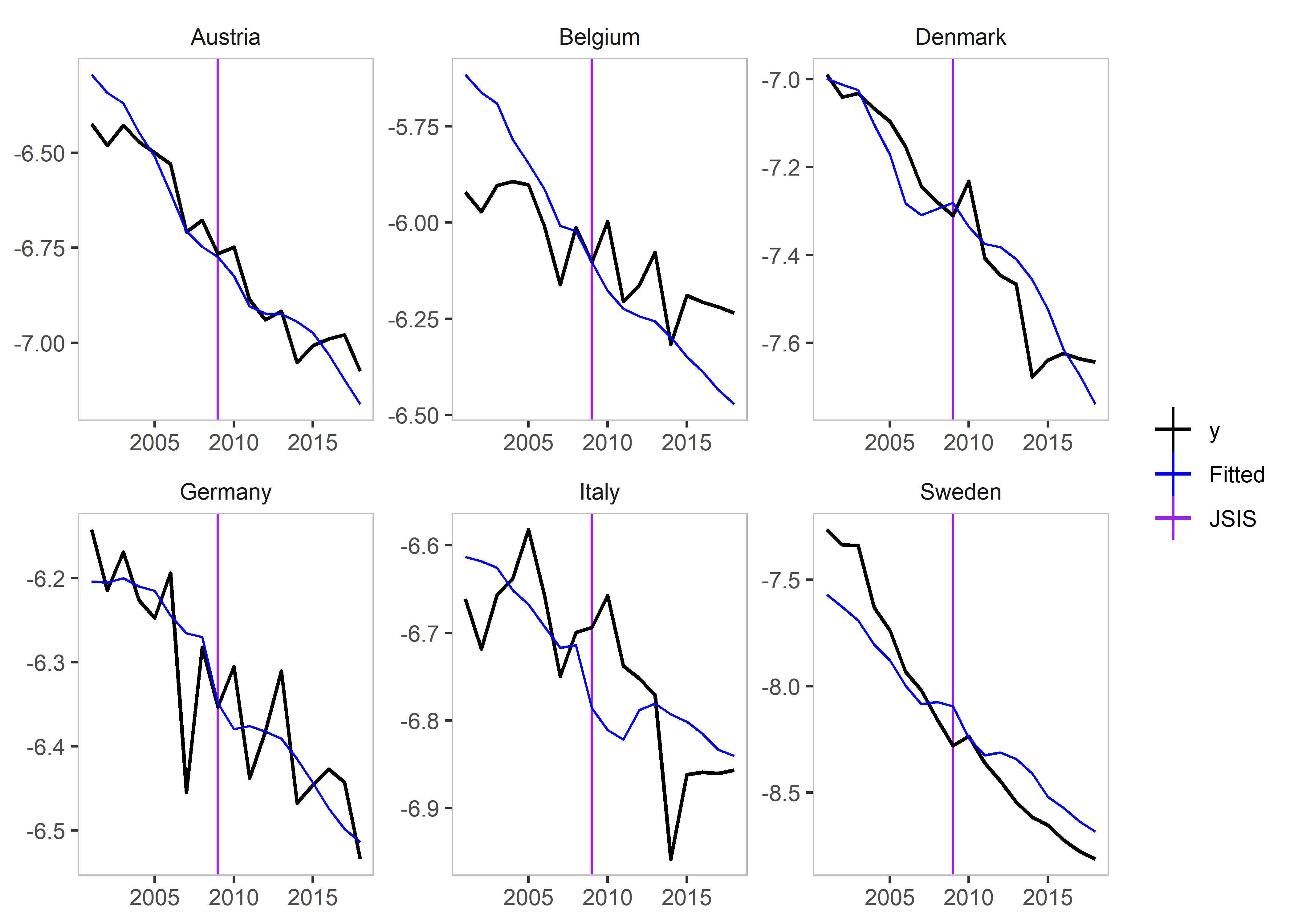### Coefficient Step Indicator Saturation

Note: This method has only been tested using the `lm` implementation (using `data`, `formula`, and `index`).

This method allows detection of coefficient breaks that are common across all groups. It is the interaction between `jsis` and the relevant coefficient.

To illustrate this, as well as the advantages of using the `lm` approach, we include a non-linear term of the lgdp variable using `I(lgdp^2)`:

``````csis_example <- isatpanel(data = EUCO2residential,
formula = lagg.directem_pc ~ lgdp + I(lgdp^2) + pop,
index = c("country","year"),

effect = "twoways",

csis = TRUE)``````
``plot(csis_example)``